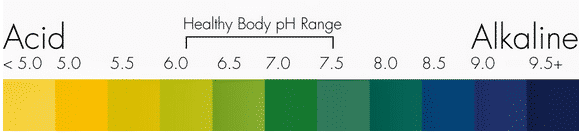FREE SHIPPING ON SUBSCRIPTIONS SHOP NOW

# What Is pH and What Does It Have To Do With WaterpH expresses a solution's relative acidity or alkalinity on a scale that ranges from 0 to 14
PH (potential of hydrogen) is the measurement of the ratio of hydrogen ions (H+) to hydroxide ions (OH-) in a solution that expresses its relative acidity or alkalinity on a scale that ranges from 0 to 14. The higher the concentration of hydrogen ions in a solution, the lower its pH, and vice versa. A pH of 7 is neutral. Anything below 7 is acidic, and anything above 7 is alkaline. Water is actually at the core of the concept of pH, since in its purest form it is the basis for a neutral pH value. This is because pure water is composed of equal parts hydrogen ions (H+) and hydroxide ions (OH-). The positively charged hydroxide ion bonds to the negatively charged hydroxide ion in a one-to-one ratio, and the two effectively neutralize each other for a pH of 7. Since pH is calculated on a logarithmic scale, the difference between each pH value actually reflects a tenfold increase or decrease in the relative hydrogen ion concentration. A solution with a pH of 3 is ten times more acidic than one with a pH of 4 and 100 times more acidic than one with a pH of 5. A one-unit difference on the pH scale actually represents a huge change in alkalinity or acidity.

## Does All Water Have a Neutral pH?

Not even close. The water used to determine neutrality on the pH scale is pure, meaning it consists only of hydrogen and hydroxide ions. The water that we drink, which is sourced from rivers, lakes and underground aqueducts, contains any combination of dissolved minerals, gases and trace chemicals, which alter the hydrogen/hydroxide ratio and therefore change the pH. This is why the water that we drink ranges on the pH scale and can be either alkaline or acidic.

Waiakea Nui

1 Liter

Waiakea Nunui

1.5 Liter

Waiakea Iki

500ML